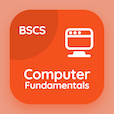Online CS Degree Courses

Digital Logic Design Quizzes

Digital Logic Design Quiz - Complete

# Transition Table in Logic Design MCQ Questions PDF Download - 107

Study Transition Table in Logic Design Multiple Choice Questions and Answers (MCQs), transition table in logic design quiz answers PDF, test 107 to download Digital Logic Design book. Asynchronous Sequential Logic MCQ trivia questions, transition table in logic design Multiple Choice Questions (MCQ Quiz) for online college degrees. Transition Table in Logic Design Book PDF: axiomatic definition of boolean algebra, flip-flops excitation tables, flip-flops, introduction to algorithmic state machine, transition table in logic design test prep for associates in computer science.

"The table having one state in each row is called " Quiz PDF: transition table in logic design App APK with state table, transition table, flow table, and primitive flow table choices for top computer science schools. Learn asynchronous sequential logic questions and answers to improve problem solving skills for online degrees.

## Transition Table in Logic Design MCQ Questions

MCQ: The table having one state in each row is called

transition table
state table
flow table
primitive flow table

MCQ: A method used to specify the sequence of the algorithm is

map
data
flowchart
operation

MCQ: Which one of the following is an example of the use of an S-R flip-flop?

transition pulse generator
racer
switch debouncer
astable oscillator

MCQ: The table that lists the inputs for the required change of states is called

truth table
excitation table
state table
clock table

MCQ: X.x' is equal to

0
1
x
x'

### More Quizzes from Digital Logic Design Book